Latest Banking jobs   »   Quantitative Aptitude Quiz For SBI Clerk...

# Quantitative Aptitude Quiz For SBI Clerk Mains 2021- 6th August

Direction (1 – 5): What will come in the place of question(?) mark in given number series:
Q1. 55, 72, 91, 112, 135, ?
(a) 156
(b) 160
(c) 144
(d) 164
(e) 172

Q2. 12.5, 13, 27.5, 85, 343.5, ?
(a) 1722
(b) 1740
(c) 1720
(d) 1716
(e) 1748

Q3. 1783, 1776, 1805, 1744, 1873, ?
(a) 1652
(b) 1668
(c) 1664
(d) 1680
(e) 1662

Q4. 12, 91, 552, 2765, 11064, ?
(a) 33180
(b) 33210
(c) 33195
(d) 33200
(e) 33560

Q5. 5856, 488, 4880, 610, 3660, ?
(a) 915
(b) 905
(c) 918
(d) 920
(e) 924

Directions (6-10): In the given questions, two quantities are given, one as ‘Quantity I’ and another as ‘Quantity II’. You have to determine relationship between two quantities and choose the appropriate option:

Q6. Quantity I – A can complete a task in 24 days and B can do same task in 18 days. another two persons C & D complete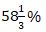of the same task in 7 days and efficiency of D is 40% more than that of C. Find in how many days A, B and D will be complete the task together?
Quantity II – Ankit is 60% less efficient than Satish and complete a piece of work in 22.5 days. Ankit and Saatish start work together and after 4.5 days both left the work, if veer complete the remaining of work in 4.5 days then find in how many days the whole work will be completed, if all three work together ?
(a) Quantity I > Quantity II
(b) Quantity I < Quantity II
(c) Quantity I ≥ Quantity II
(d) Quantity I ≤ Quantity II
(e) Quantity I = Quantity II or no relation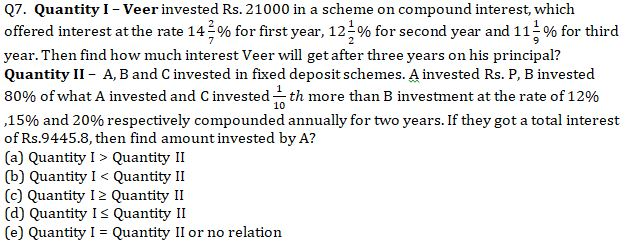Q8. Quantity I – A shopkeeper has two articles A & B. Marked price of article B is 20% more than marked price of article A, shopkeeper sold article A at 25% discount and article B at 20% discount. He made 20% loss on article A and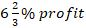profit on article B. If total loss of shopkeeper was Rs. 765, then find marked price of article B?
Quantity II – A shopkeeper gives a discount of 24% on marked price of Shirt and cost price of Jeans is 25% more than selling price of Shirt. If shopkeeper sold Jeans at 10 % profit and selling price of Jeans was Rs. 1140 more than selling price of Shirt , then find the cost price of article Jeans ?
(a) Quantity I > Quantity II
(b) Quantity I < Quantity II
(c) Quantity I ≥ Quantity II
(d) Quantity I ≤ Quantity II
(e) Quantity I = Quantity II or no relation

Q9. Quantity I – A bag contains four green balls, three red balls and five blue balls. If three balls taken out at random what is probability of at least one ball is green and at least one ball is blue color.
Quantity II – There are five red toys and six green toys in a cartoon. What will be the probability of selection of four toys which contains at least two green toys?
(a) Quantity I > Quantity II
(b) Quantity I < Quantity II
(c) Quantity I ≥ Quantity II
(d) Quantity I ≤ Quantity II
(e) Quantity I = Quantity II or no relation

Q10. Quantity I – Six years ago ratio between age of Rohit and Prakash was 7 : 8, while six years hence ratio between 1/6 th of Rohit age and 1/3 rd of Prakash age will be 9 : 20. The age of Rohit two years hence will be.
Quantity II – Ratio between age of A, B and C is 16 : 9 : 7. Three years hence average of all three age will be 35 years. The age of A two years hence will be?
(a) Quantity I > Quantity II
(b) Quantity I < Quantity II
(c) Quantity I ≥ Quantity II
(d) Quantity I ≤ Quantity II
(e) Quantity I = Quantity II or no relation

Q11. B is thrice as efficient as C. B and C can compete a work together in 45/2 days. A takes 50% more days than the days taken by A and B to complete the same work together.
Quantity 1: No. of days taken by fastest among them to complete the work alone.
Quantity 2: Time taken by A and C to complete the work together.
(a) Quantity I > Quantity II
(b) Quantity I < Quantity II
(c) Quantity I ≥ Quantity II
(d) Quantity I ≤ Quantity II
(e) Quantity I = Quantity II or No relation

Q12. Quantity I→ The profit earned by selling an item(in Rs) if the difference b/w the SP and the CP is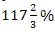of 600.
Quantity II→ The cost price of an article (in Rs) if the selling price of the article is 1000 Rs and he got 25% profit after selling the item.
(a) Quantity I > Quantity II
(b) Quantity I < Quantity II
(c) Quantity I  Quantity II
(d) Quantity I = Quantity II
(e) No relation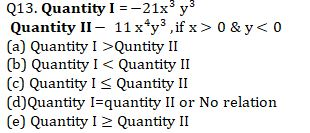Q14. The largest possible right circular cylinder is cut out from a wooden cube of edge 7 cm.
Quantity I: volume of the cube left over after cutting out the cylinder
Quantity II: Surface area of cube remained after cutting out the cylinder.
Note: compare the magnitudes of both quantities.
(a) Quantity I > Quantity II
(b) Quantity I < Quantity II
(c) Quantity I ≥ Quantity II
(d) Quantity I ≤ Quantity II
(e) Quantity I = Quantity II or No relation

Q15. Quantity I: difference between the largest and the smallest sum. A sum of Rs. 1440 is lent out in three parts in such a way that the interests on first part at 2% for 3 years, second part at 3% for 4 years and third part at 4% for 5 years are equal.
Quantity II : 460
(a) Quantity I > Quantity II
(b) Quantity I < Quantity II
(c) Quantity I ≥ Quantity II
(d) Quantity I ≤ Quantity II
(e) Quantity I = Quantity II or No relation

Solution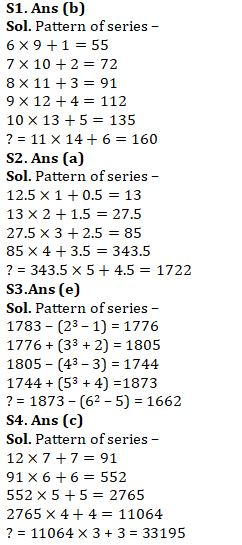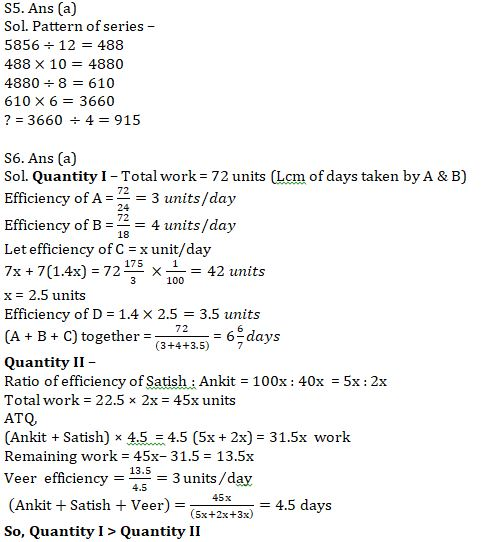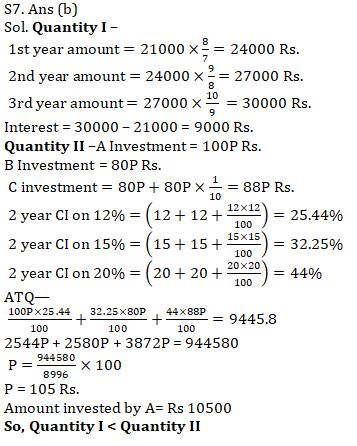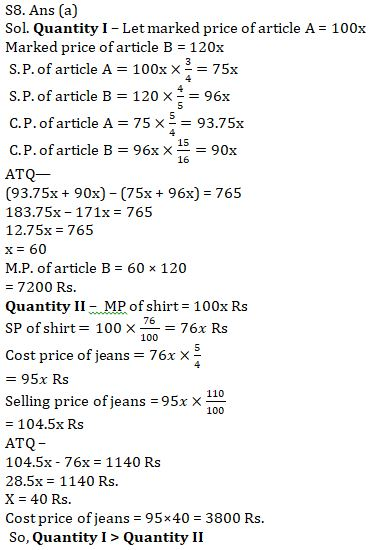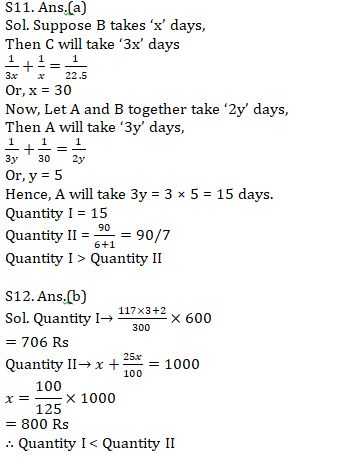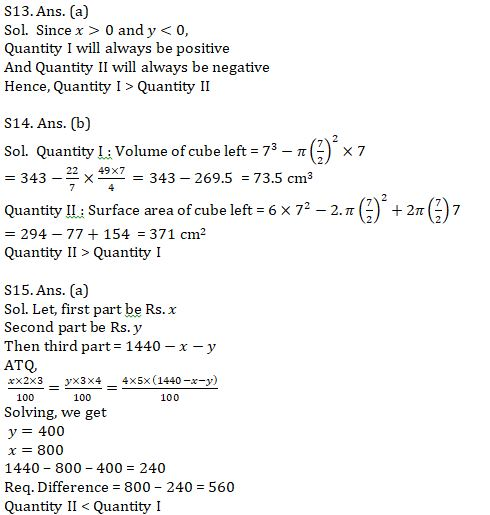#### Congratulations!Union Budget 2023-24: Free PDF